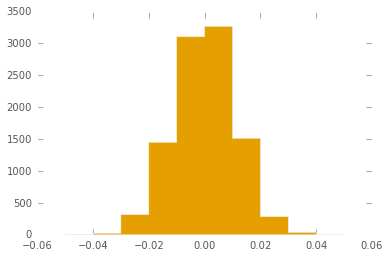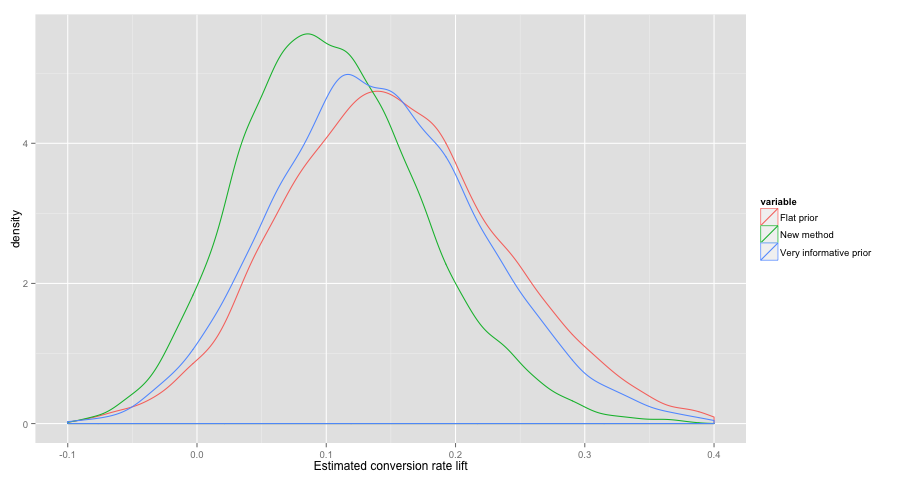# Update 2014-10-15

Once again my answer is too complicated. Regularized logistic regression is a similar model which gives pretty much the same answer. Update discussion.

# Intro¶

The typical approach to Bayesian AB testing doesn't really allow for prior knowledge on the scale of the change in conversion rate. Sometimes you know that a change will probably have only a small effect. I propose a slightly different approach that explicitly models knowledge about the expected effect size.

# The usual way of thinking about it¶

We have two different versions of a website, A and B, and we wish to estimate their true conversion rates $p_A$ and $p_B$. (Or more likely we want an estimate of the difference or ratio of the conversion rates, with some notion of the uncertainty around that estimate.) We observe a number of visits, some of which convert, to each version of the site. Call these $obs_A$ and $obs_B$. We also have some prior knowledge of the possible values for $p_A$ and $p_B$ which we model as beta distributions $B_A$ and $B_B$. A typical choice for these is the flat, uniform prior for each: $B(1,1)$.

So the data story goes like this for each variation:

1. Select the conversion rate $p$, where $p$ is beta distributed according to the prior $B$.
2. Observe a number of visits which are Bernoulli distributed with rate $p$.
$$B \rightarrow p \rightarrow obs$$

# A problem with this story¶

Often the differences between A and B are small, e.g. rephrasing the call to action on a button. So you have prior knowledge that the difference in conversion rates $p_A$ and $p_B$ is probably small. The above story doesn't really allow you to use that knowledge. The problem is worse the flatter your priors $B_A$ and $B_B$ are. In the case of a uniform prior for both, we're saying that $p_A, p_B = 0\%, 100\%$ is equally likely to $p_A, p_B = 25\%, 25\%$, which is crazy for a small change.

# A better data generation story¶

I use an alternative parameterization of the beta distribution, based on the mean $\mu = \frac{\alpha}{\alpha+\beta}$ and "sample size" or spread $\tau = \alpha + \beta$.

Rather than using a fixed beta prior for A and B, we allow it to vary according to our prior knowledge. The mean $\mu$ varies according to historical data, much like in the usual approach. Denote the varying beta prior $B_{\mu}$ to indicate it's dependence on $\mu$. Fix the spread $\tau$ to a value which is consistent with our beliefs about the possible effect size. $p_A$ and $p_B$ are then drawn from this beta distribution.

The story looks like this:

1. Based on historical data, select a beta distribution $B_0$
2. Draw a $\mu$ from $B_0$
3. Based on intuition/experience, choose a spread parameter $\tau$
4. We now have a beta distribution $B_{\mu}$
5. Select $p_A$ and $p_B$ from $B_{\mu}$
6. Observe visits that are Bernoulli distributed according to $p_A$ and $p_B$

In picture form:# Tuning $\tau$¶

The step for coming up with $\tau$ sounds a bit magical in the above description, so let's be more concrete. Suppose that from our historical data, the mean conversion rate for situations similar to our current experiment is 10%, so $\mu=.1$. Setting $\tau=1500$ then produces a distribution of the conversion rate difference shown below. Increasing $\tau$ narrows the distribution. Use a bit of guess and check to come up with something reasonable here.

In :
import numpy as np
from scipy.stats import beta as beta_dist
%matplotlib inline

In :
mu = .10
tau = 1500
alpha, beta = mu*tau, (1-mu)*tau
pA = beta_dist.rvs(alpha,beta,size=10000)
pB = beta_dist.rvs(alpha,beta,size=10000)
diff = Series(pA-pB)
diff.hist(bins=np.arange(-.05,.06,.01), histtype='stepfilled')
print(diff.describe())

count    10000.000000
mean         0.000137
std          0.010988
min         -0.042673
25%         -0.007296
50%          0.000271
75%          0.007532
max          0.037420
dtype: float64# Example¶

In the example below, it's early in the AB test and we only have a few thousand sessions. But the results look very promising, almost a 15% lift in the empirical conversion rate. But how realistic is that, if it was only a small change to the website? Should we stop the test and call it a win?

I take a look at the estimated percentage improvement in conversion rate with the usual method versus the new method. For the usual method, I try it with a flat prior $B(1,1)$ as well as the very informative prior $B(30, 270)$.

The new method gives a much more modest estimate of the improvement.

In :
successesA = 300
successesB = 350
failuresA = 3000
failuresB = 3000
pA, pB = successesA/(successesA+failuresA), successesB/(successesB+failuresB)
pA, pB, (pB-pA)/pA

Out:
(0.09090909090909091, 0.1044776119402985, 0.1492537313432835)
In :
def usual_method(alpha=1, beta=1):
pA = beta_dist.rvs(alpha+successesA,beta+failuresA,size=10000)
pB = beta_dist.rvs(alpha+successesB,beta+failuresB,size=10000)
lift = Series((pB-pA)/pA)
return lift

In :
def new_method(alpha0=3, beta0=27, tau=1500):
mu_samples = beta_dist.rvs(alpha0, beta0, size=10000)
def compute_p(mu):
alpha, beta = mu*tau, (1-mu)*tau
pA = beta_dist.rvs(alpha+successesA,beta+failuresA)
pB = beta_dist.rvs(alpha+successesB,beta+failuresB)
return pA, pB
p = np.array([compute_p(mu) for mu in mu_samples])
pA, pB = p[:, 0], p[:, 1]
lift = Series((pB-pA)/pA)
return lift

In :
lift0 = usual_method()
lift1 = usual_method(30, 270)
lift2 = new_method()
df = DataFrame({'Flat prior': lift0, 'Very informative prior': lift1, 'New method': lift2})
df = pd.melt(df)


If we're using a simple decision rule like "stop the test if one variation has a 95% or greater of being the best" then the usual method would stop here, but the new method would want to gather more data:

In :
(lift0<0).mean(), (lift1<0).mean(), (lift2<0).mean()

Out:
(0.031800000000000002, 0.035200000000000002, 0.059999999999999998)

Even using an unrealistically informative prior doesn't shift our estimated CVR lift too much. But the new method does.

In :
%load_ext rmagic

In :
%%R -i df -w 900
library(ggplot2)
p <- ggplot(df, aes(x=value, color=variable)) + geom_density()
p + xlab('Estimated conversion rate lift') + xlim(-.1, .4)